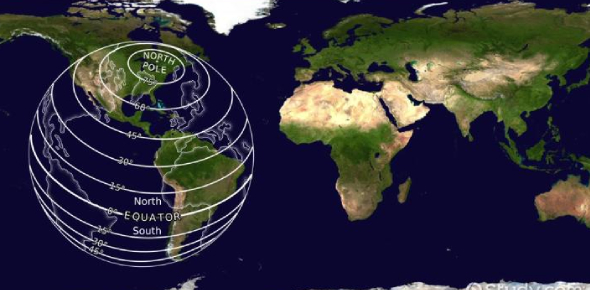# Lake Effect-longitude And Latitude

10 Questions | Total Attempts: 1046SettingsPlease take the quiz based on the lesson and activities that we have completed together in school.

• 1.
Latitude and longitude are measured in?
• A.

Miles

• B.

Kilometers

• C.

Degrees

• D.

Hours

• 2.
Which lines run north and south along the earth's surface? Choose all that correct answers that apply?
• A.

Latitude lines

• B.

Longitude lines

• C.

Equator

• D.

Prime meridian

• 3.
The highest number of degrees in latitude is 90?
• A.

True

• B.

False

• 4.
Lines of latitude can be thought of like telephone poles?
• A.

True

• B.

False

• 5.
The intersection of a particular latitude line with a given longitude line is known as?
• A.

Coordinate

• B.

Absolute location

• C.

Interloping

• D.

Relative location

• 6.
Degrees of latitude and longitude can be divided into: Choose all that correct answers that apply?
• A.

Hours

• B.

Minutes

• C.

Seconds

• D.

Days

• 7.
When finding longitude, what is your first step?
• A.

Determine the direction

• B.

Locate the equator

• C.

Determine the distance

• D.

Go to the starting line

• 8.
Longitude tells you the distance east or west of the Prime Meridian?
• A.

True

• B.

False

• 9.
What are some of the ways in which we use latitude and longitude in everyday life? Choose all that correct answers that apply?
• A.

To aim missles in the military

• B.

To locate the survivors of a plane crash

• C.

To locate the survivors of a plane crash

• D.

To determine the height of a skyscraper

• 10.
When stating the absolute location of a place, you always give the longitude first?
• A.

True

• B.

False

Related TopicsBack to top